# Uncertainties Module 4 - short version

Uncertainty Module 4 - UM Student Guide Feb. 16, 2018, 11:58 a.m.

1. Introduction to Gaussian Distributions

In Modules 2 and Module 3 we considered a single measurement of some physical quantity. In each of the examples we discussed, repeating the measurement of the same object using the same instrument almost certainly would give the same result. So repeating these measurements doesn’t give us any added information about the value and uncertainty of the quantity being measured. In this Module we will think about cases where repeated measurements do not give the same value of the measurand, and you will measure the time for a piece of paper to fall to the floor.

We will begin by thinking about the following experimental apparatus.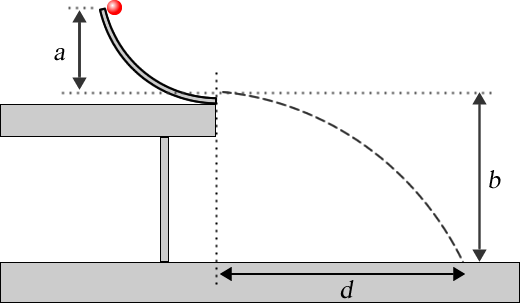#### Figure 1

A curved ramp is mounted on a table. You release a small ball from rest at the top of the ramp, it rolls down the ramp, and then travels along the dashed path. It the absence of air resistance and for a very small ball, Newton’s Laws can be used to show that the theoretical value of d, dtheory is:

$$d_{\rm theory}=2\sqrt{ab}$$      (1)

In your actual experiment, there is a special paper on the floor where the ball lands and when the ball strikes the paper it will leave a mark on it where it landed. We measure the horizontal distance d the ball travels when it hits the floor. It is hard for you to release the ball from exactly the same position each time, and the ramp and ball are not completely smooth so the ball bounces around a bit as it goes down the ramp. Therefore, if you repeat the measurement a few times, it is unlikely that the ball landed in exactly the same place each time. Perhaps after 5 trials the paper looks like Figure 2. We call such measurements scattered or dispersed.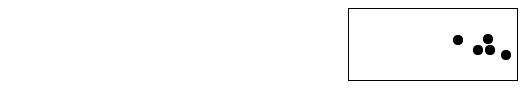#### Figure 2

Bell-shaped curves are often called Gaussian distributions because Carl Friedrich Gauss studied them extensively in the early 19th century. They occur so often that sometimes they are called normal distributions. We can write a formula for the amplitude n(x) of a bell-shaped curve for a variable x as:

$$n(x)=n_{\rm max}e^{-{(x-\mu)^2 \over 2\sigma^2}}$$      (2)

where nmax is the maximum value of n$$\mu$$ is the value x for which n(x) = nmax and $$\sigma$$ is the standard deviation. As you will soon see, this is the same standard deviation you learned about in Module 2 and Module 3.

We measure the horizontal position xi for each of the i trials with a ruler. For now we will ignore the uncertainty in the measurement by the ruler: instead we will concentrate on the spread of values that we see in Figure 2.

##Question 1

Imagine that the data of the experiment in Figures 1 and 2 gives distances d between 0.62 and 0.73 m. True Gaussians, Eqn. 2, only approach zero asymptotically as $$x \rightarrow \pm \infty$$ . So if we use a Gaussian probability distribution function (Equation 2) to describe the data of experiment of Figure 1, this says that there is a small but non-zero probability of getting a result of $$d=-432\ {\rm km}$$. Is this physically possible? What does this tell you about using a Gaussian pdf?

For a finite number of measurements we can estimate the mean:

$$\bar{x} = {1 \over N}\sum \limits_{i=1}^N x_i\$$       (3)

We can also use the data to estimate the standard deviation:

\begin{align*} \sigma & = \sqrt{ {1 \over N-1} \sum_{i=1}^N(x_i-\bar{x})^2} \end{align*}       (4)

For any individual measurement xi, the estimated uncertainty in the value of the measurand is:

$$u(x_i)=\sigma$$      (5)

Note that this is not the uncertainty in the value of the estimated mean $$\bar{x}$$: it is the uncertainty in each individual measurand xi. For a Gaussian pdf, the area under the curve between $$\mu - \sigma$$ and  ​$$\mu + \sigma$$​ is 0.68. Therefore it is reasonable to assume that the probability that for a single measurement xi the true value of $$\bar{x}$$ is within $$\sigma$$ of xi is 0.68. Put another way, in the experiment of Figures 1 and 2 if modeling the pdf as a Gaussian is reasonable, then if you choose one of the measurements of the distance xi at random, there is a 68% chance that it is within one standard deviation of the true value of the position.

Since this uncertainty arises from the scatter of values due to various random effects, this type of uncertainty is often called statistical.

2. Significant Figures Involving Uncertainties

When uncertainties for quantities are given, the rules for significant figures are:

1. Uncertainties should be specified to one, or at most two significant figures.
2. The most precise column in the number for the uncertainty should also be the most precise column in the number for the value.

So if the uncertainty is specified to the 1/100th column, the quantity itself should also be specified to the 1/100th column.

##Question 2

Express the following quantities to the correct number of significant figures:

1. 25.052 ± 1.502
2. 92 ± 3.14159
3. 0.0530854 ± 0.012194
4. $$3.2478 \times 10^{-6} \pm 1.9518 \times 10^{-7}$$
5.  $$6.674076391 \times 10^{-11} \pm 3.10895 \times 10^{-15}$$You may have seen other definitions and ways of dealing with significant figures elsewhere. For experimentally determined quantities, those definitions and properties are not appropriate! Use these rules instead!

3. Propagation of Uncertainties

Say we have measured some quantity x with uncertainty u(x) and a quantity y with uncertainty u(y) and wish to combine them to get a value z with uncertainty u(z). As we discussed in Module 2, we need the combination to preserve the probabilities associated with the uncertainties in x and y. We will consider a number of ways of combining the quantities. Although this Module has been discussing statistical uncertainties, this section applies to all uncertainties, including the ones you learned about in Module 2 and Module 3.

As discussed in Module 2 and Module 3, if z = x + y or z = xy then the uncertainties are combined in quadrature:

$$u(z)=\sqrt{u(x)^2 + u(y)^2}$$       (6)

#### Multiplication or Division

If z = xy or z = x/y then the fractional uncertainties are combined in quadrature:

$$\begin{eqnarray*} {u(z) \over |z|} = \sqrt{\left({u(x) \over x}\right)^2 + \left({u(y) \over y}\right)^2} \end{eqnarray*}$$​       (7)

#### Multiplication by a Constant

If z = ax, where a is a constant known to a large number of significant figures, then the uncertainty in z is given by Eqn. 7 with the uncertainty in a, u(a) = 0. So:

$$u(z) = |a| u(x)$$      (8)

#### Raising to a Power

If z = xn then:

$$u(z) = n x^{(n-1)}u(x)$$      (9)

which can also be written in terms of the fractional uncertainties:

$$\begin{eqnarray*} {u(z) \over z} = n{u(x) \over x} \end{eqnarray*}$$     (10)Say you are squaring x, so $$z = x^2 = x \times x$$. You may be tempted to use Eqn 7 for multiplication and division, but this is incorrect: Eqn 7 assumes that the uncertainties in the quantities x and y are independent of each other. Here there is only one quantity, x.

Be sure to remember that in call cases u(z) defines the significant figures in u.

#### The General Case

In general z is some function of x and y, z = f(x, y). The uncertainty in z is given by partial derivatives:

$$u(z)=\sqrt{\left[ {\partial f(x,y) \over \partial x}u(x)\right]^2+\left[ {\partial f(x,y) \over \partial y}u(y)\right]^2}$$      (11)

Eqns. 6 – 10 are just applications of Eqn. 11 for various functions.

##Question 3

Eqn. 9 may look familiar to you. What does it look like? Hint: try writing u(z) as dz and u(x) as dx.

##Question 4

You measure a quantity to be $$3 \pm 1$$ and another quantity to be $$70 \pm 2$$ . What is the uncertainty in the sum to one significant figure? Does the uncertainty in the value of 3 have any effect on the uncertainty in the sum to one significant figure? Write down the sum $$\pm$$ its uncertainty to the correct number of significant figures. Remember that the uncertainty only has one or at the very most two digits that really are significant, and that the uncertainty determines the number of digits in the value that are significant.

4. The Uncertainty in the Mean

We have seen that for N repeated measurements, x1, x2, … , xN, the statistical uncertainty in each individual measurand xi is the standard deviation $$\sigma$$. We now know enough to determine the uncertainty in the estimated mean, $$u(\bar{x})$$. The estimated mean is given by:

\begin{align*} \bar{x} & = {1 \over N} \sum_{i=1}^N x_i \\ & = { [x_1 \pm u(x_1)] + [x_2 \pm u(x_2)] + ... + [x_N \pm u(x_N)]\over N} \end{align*}     (12)

But the uncertainty in each individual measurement is the same, which we will call u(x): $$u(x) \equiv u(x_1) = u(x_2) = ... = u(x_N)$$. Combining all the uncertainties in the numerator in quadrature gives:

$$\begin{eqnarray*} \bar{x} = { (x_1 + x_2 + ... + x_N) \pm \sqrt{N} u(x) \over N} \end{eqnarray*}$$      (13)

The numerator is divided by the constant N, so from Eqn. 7:

​$$\begin{eqnarray*} \bar{x} = { (x_1 + x_2 + ... + x_N) \over N} \pm { u(x) \over \sqrt{N}} \end{eqnarray*}$$​     (14)

or:

$$\begin{eqnarray*} u(\bar{x})={u(x) \over \sqrt{N} } \end{eqnarray*}$$     (15)

So repeating a measurement N times reduces the statistical uncertainty in the mean by a factor of $$1 / \sqrt{N}$$ times the uncertainty in each individual measurement. So repeating a measurement 4 times reduces the uncertainty by a factor of ½.

The fact that the uncertainty in the mean is less than the uncertainty in each individual measurement should not be a surprise: we repeat measurements precisely so that we increase our knowledge of the true value of what we are measuring, i.e. in order to reduce its uncertainty.

If we were actually doing the experiment of Figure 1, we finally could now determine if the measured value of the distance is within experimental uncertainties of the theoretical value of Eqn. 1.

5. Activities

##Activity 1

Imagine that you have measured the time for a pendulum to undergo five oscillations, t5, with a digital stopwatch. You repeat the measurements 4 times, and the data are:

 t5 (s) 7.53 7.38 7.47 7.43

What is the mean of the four measurements of t5,  and uncertainty in this mean value? Express your final result as $$\bar{t_5} \pm u(\bar{t_5})$$ .  Be sure to use the rules for significant figures when uncertainties are involved.

##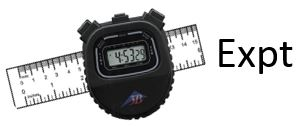Activity 2

Using the supplied digital stopwatch, try to start it and then stop it at exactly 2.00 s. Practice a few times before beginning to take the data. After practicing, repeat a few times. All members of the Team should do this, so you may end up with about 15 or 20 values. Just by looking at the data and without doing any calculations, choose a value of u such that most but not necessarily all measurements are between 2.00 – u and 2.00 + u.

##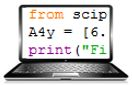Activity 3

[For this activity, it is a good idea to use Python to enter your data as you take it, just as you did for rolling dice in Module 1. It is probably an excellent idea to review how you used Python in that Module now.]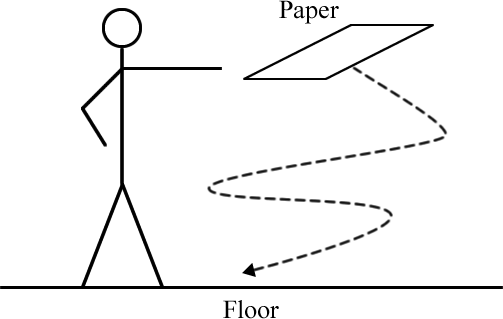You are supplied with a standard 8 ½ by 11 inch sheet of paper and a digital stopwatch. Hold the paper horizontally at shoulder height and release it. Measure the time t it takes the paper to reach the floor. Repeat for a total of 20 times, excluding trials where the paper strikes something as it falls.

Make a histogram of the results of your experiment. You will need to decide the range and how many bins to use in making the histogram. The decision is based somewhat on the scatter of values.

Is it reasonable to assume that the scatter of values of t can be described by a Gaussian probability distribution function? If not, can you think of another simple function that better describes the shape of the histogram? What is that shape, and why is it better?

What is the estimated statistical uncertainty in each measurement of t, i.e. the estimated standard deviation? The Python function to calculate standard deviations is std(). However, just as for the var() function you used in Module 1 to calculate the variances, by default the Python standard deviation function divides the N, not N – 1. So, just as for the variances, you will need to calculate std( data, ddof = 1).

In Activity 2 you estimated an uncertainty in the individual time measurements due to human reaction times, call it $$u_{\rm reaction}(t_i)$$. You have just found another uncertainty in the individual measurements, the one due do the random fluctuations in the times you measured for different trials; we will call this the statistical uncertainty ​$$u_{\rm statistical}(t_i)$$​ . It is reasonable to combine these two uncertainties in quadrature, the square root of the sum of the squares, to estimate the total uncertainty in each individual measurement.

Do the calculation of combining these two uncertainties. Remember from Question 3 that if one uncertainty is much smaller than the other, than when combining them in quadrature to only 1 or 2 significant figures the smaller value has negligible effect on the combination, and sometimes it is not even worth the effort of doing the calculation. Does the smaller of the uncertainties being combined here have a significant effect on the value of the combination?

Can you think of any other uncertainties, such as the reading uncertainty of a digital instrument or the accuracy of the stopwatch, which might have a significant effect on the total uncertainty in your measurements of ti? If so, calculate their effects.

Finally, what is estimated mean time for the paper to reach the floor, and what is the uncertainty in this time? Present your final result as $$\bar{t} \pm u(\bar{t})$$ .

##Activity 4

This activity is not about the main topic of this Module, which is repeated measurements of the same quantity. Instead it is about uncertainties in measurements using analog instruments, which you learned about in Module 3, and propagation of uncertainties when the directly measured quantities are being divided, which you have learned about in this Module.

You are supplied some circular metal hoops of different sizes. For each hoop determine its diameter and its circumference with the supplied meter stick, and include the uncertainties in your determination of the diameter and circumference. A nice way to determine the circumference is to roll the hoop on the tabletop for exactly one revolution and measure how far it rolled.

Then, for each hoop calculate the circumference divided by the diameter, and the uncertainty in the ratio. Is the ratio the same value within the calculated uncertainties for all the hoops? Is there some theoretical value of the ratio? If so, what is it and are your measurements within uncertainties of this value? Also if so, if you repeated the measurement for a large number of hoops of different sizes, would you expect all of the calculated ratios to be within uncertainties of this value, and if not what fraction of them should be within uncertainties of the theoretical value?

Based on a guide originally written by David M. Harrison, Dept. of Physics, Univ. of Toronto, September 2013.